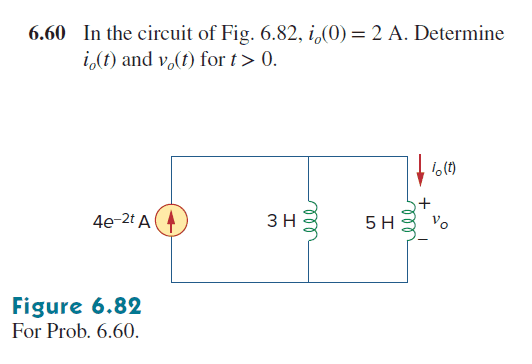# How does the current divide in parallel inductors?

jangchen
Homework Statement:
In the circuit of Fig. 63.82 io(0)=2mA. Determine io(t) and Vo(t) for t>0
Relevant Equations:
$$V_O = L*\frac{di}{dt}$$I apologize using English fluently because I am not an Enlgish speaker.

When I tried to solve this problem, I used current divider rule.

So, $$i_o(t) = \frac{3}{3+5}*4e^{-2t} = 1.5*e^{-2t} A$$

However, This was wrong.

The answer is $$1.5*e^{-2t} + 0.5 A$$

If I use $$V_O = L*\frac{di}{dt}$$ , I can get right answer.

I wonder why there is a difference
between using current divider rule and using $$V_O = L*\frac{di}{dt}$$.

•Delta2

Gold Member
The current divider rule assumes a steady state solution. This is a transient problem. The current divider rule doesn't properly account for the initial conditions. At t=0 each inductor has 2A, which isn't consistent with the 3:5 ratio.

Homework Helper
The answer is $$1.5*e^{-2t} + 0.5 A$$

If I use $$V_O = L*\frac{di}{dt}$$ , I can get right answer.

I wonder why there is a difference
between using current divider rule and using $$V_O = L*\frac{di}{dt}$$.
The voltage divider rule is valid for resistors, when the voltage is proportional to the current, U=RI. In case of inductors, this is not true, U=LdI/dt instead.

•jangchen and Delta2
jangchen
The current divider rule assumes a steady state solution. This is a transient problem. The current divider rule doesn't properly account for the initial conditions. At t=0 each inductor has 2A, which isn't consistent with the 3:5 ratio.